# NLP重大突破？一文读懂XLNet“屠榜”背后的原理

## 语言模型和BERT各自的优缺点

$\underset{\theta}{max}\; log p_\theta(\mathbf{x})=\sum_{t=1}^T log p_\theta(x_t \vert \mathbf{x}_{<t})=\sum_{t=1}^T log \frac{exp(h_\theta(\mathbf{x}_{1:t-1})^T e(x_t))}{\sum_{x'}exp(h_\theta(\mathbf{x}_{1:t-1})^T e(x'))} \text{ 等式(1)}$

$\underset{\theta}{max}\;log p_\theta(\bar{\mathbf{x}} | \hat{\mathbf{x}}) \approx \sum_{t=1}^Tm_t log p_\theta(x_t | \hat{\mathbf{x}})=\sum_{t=1}^T m_t log \frac{exp(H_\theta(\mathbf{x})_{t}^T e(x_t))}{\sum_{x'}exp(H_\theta(\mathbf{x})_{t}^T e(x'))} \text{ 等式(2)}$

• 独立假设

• 注意等式(2)的约等号$\approx$，它的意思是假设在给定$\hat{\mathbf{x}}$的条件下被Mask的词是独立的(没有关系的)，这个显然并不成立，比如"New York is a city"，假设我们Mask住"New"和"York"两个词，那么给定"is a city"的条件下"New"和"York"并不独立，因为"New York"是一个实体，看到"New"则后面出现"York"的概率要比看到"Old"后面出现"York"概率要大得多。而公式(1)没有这样的独立性假设，它是严格的等号。
• 输入噪声

• BERT的在预训练时会出现特殊的[MASK]，但是它在下游的fine-tuning中不会出现，这就是出现了不匹配。而语言模型不会有这个问题。
• 双向上下文

• 语言模型只能参考一个方向的上下文，而BERT可以参考双向整个句子的上下文，因此这一点BERT更好一些。关于为什么RNN只能是单向的上下文而BERT可以参考整个句子的上线，读者可以参考ELMo和OpenAI GPT的问题

## 排列(Permutation)语言模型

$p(\mathbf{x})=p(x_1)p(x_2|x_1)p(x_3|x_1x_2) \Rightarrow 1 \rightarrow 2 \rightarrow 3 \\ p(\mathbf{x})=p(x_1)p(x_2|x_1x_3)p(x_3|x_1) \Rightarrow 1 \rightarrow 3 \rightarrow 2 \\ p(\mathbf{x})=p(x_1|x_2)p(x_2)p(x_3|x_1x_2) \Rightarrow 2 \rightarrow 1 \rightarrow 3 \\ p(\mathbf{x})=p(x_1|x_2x_3)p(x_2)p(x_3|x_2) \Rightarrow 2 \rightarrow 3 \rightarrow 1 \\ p(\mathbf{x})=p(x_1|x_3)p(x_2|x_1x_3)p(x_3) \Rightarrow 3 \rightarrow 1 \rightarrow 2$

$\underset{\theta}{max} \mathbb{E}_{z \sim \mathcal{Z}_T}[\sum_{t=1}^Tlog p_\theta(x_{z_t}|\mathbf{x}_{z_{<t}})]$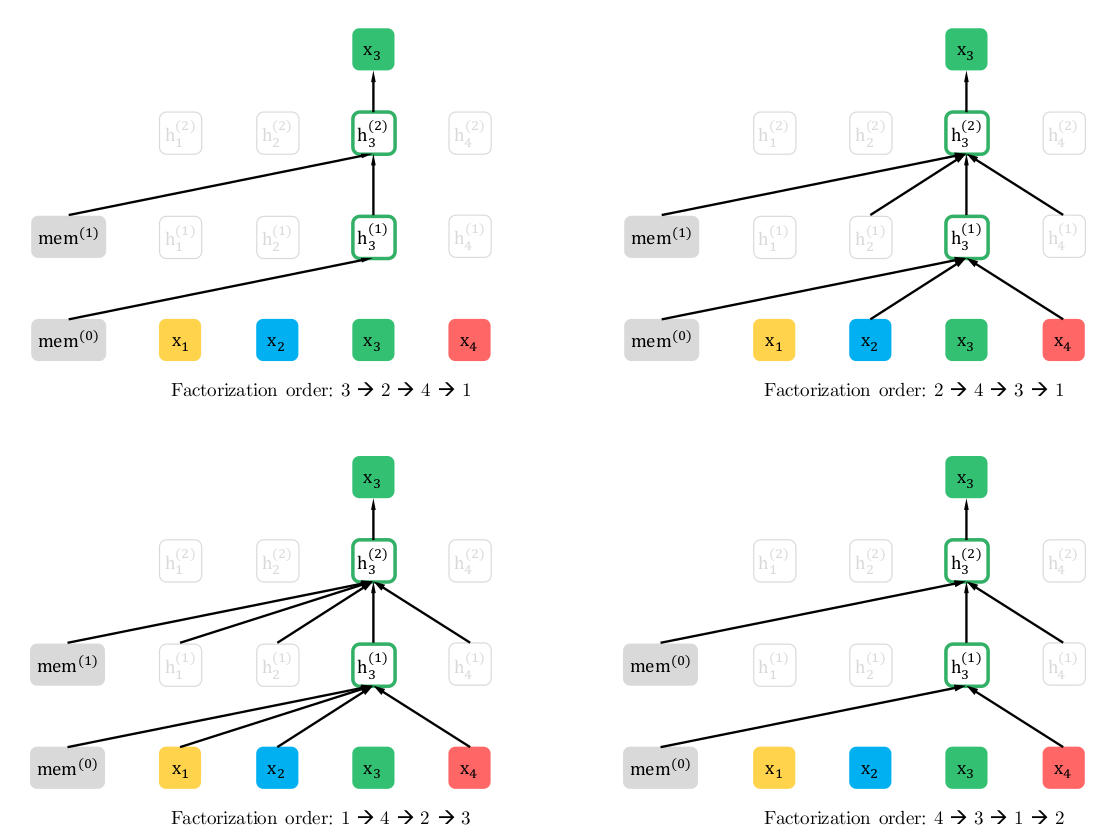## Two-Stream Self-Attention for Target-Aware Representations

### 没有目标(target)位置信息的问题

$p_\theta(X_{z_3}=x|x_{z_1z_2})=p_\theta(X_4=x|x_1x_3)=\frac{exp(e(x)^Th_\theta(x_1x_3))}{\sum_{x'}exp(e(x')^Th_\theta(x_1x_3))}$

$p_\theta(X_{z_3}=x|x_{z_1z_2})=p_\theta(X_2=x|x_1x_3)=\frac{exp(e(x)^Th_\theta(x_1x_3))}{\sum_{x'}exp(e(x')^Th_\theta(x_1x_3))}$

$p_\theta(X_{z_t}=x \vert \mathbf{x}_{z_{<t}})=\frac{exp(e(x)^Th_\theta(\mathbf{x}_{z_{<t}}))}{\sum_{x'}exp(e(x')^Th_\theta(\mathbf{x}_{z_{<t}}))}$

$p_\theta(X_{z_t}=x \vert \mathbf{x}_{z_{<t}})=\frac{exp(e(x)^Tg_\theta(\mathbf{x}_{z_{<t}}, z_t))}{\sum_{x'}exp(e(x')^Tg_\theta(\mathbf{x}_{z_{<t}}, z_t))}$

### Two-Stream Self-Attention

• 为了预测$\mathbf{x}_{z_t}$$g_\theta(\mathbf{x}_{z_{<t}}, z_t)$只能使用位置信息$z_t$而不能使用$\mathbf{x}_{z_t}$。这是显然的：你预测一个词当然不能知道要预测的是什么词。

• 为了预测$z_t$之后的词，$g_\theta(\mathbf{x}_{z_{<t}}, z_t)$必须编码了$x_{z_t}$的信息(语义)。

• 内容隐状态$h_\theta(\mathbf{x}_{z_{<t}})$，简写为$h_{z_t}$，它就会标准的Transformer一样，既编码上下文(context)也编码$x_{z_t}$的内容。

• 查询隐状态$g_\theta(\mathbf{x}_{z_{<t}}, z_t)$，简写为$g_{z_t}$，它只编码上下文和要预测的位置$z_t$，但是不包含$x_{z_t}$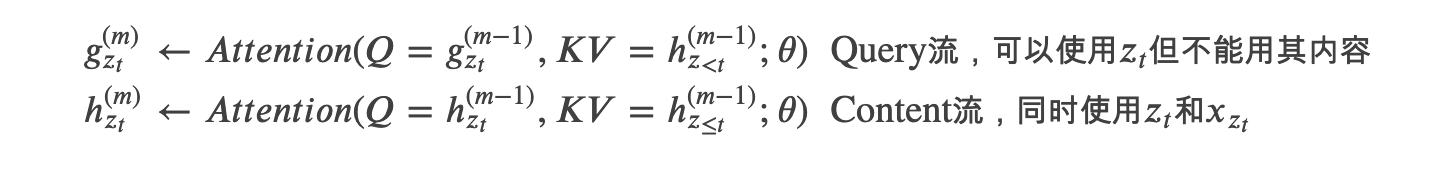上面两个流分别使用自己的Query向量$g_{z_t}$$h_{z_t}$；但是Key和Value向量都是用的h，因为h是内容。但是注意Query流不能访问$z_t$的内容，因此KV是$h_{z_{<t}}^{(m-1)}$，这里用的是小于号(<)表示不包括t时刻的content。而Content流的KV是$h_{z_{\le t}}^{(m-1)}$，它包含$x_{z_t}$## 部分预测

$\frac{|z|-c}{|z|}=\frac{1}{K}$

## 融入Transformer-XL的优点

### Transformer-XL思想简介

• 由于定长的要求，我们不可能让输入太长。因此虽然Self-Attention机制虽然不太受长度的约束，但是Transformer的语言模型实际能够考虑的上下文就是输入的长度。

• 因为我们在序列语言模型的时候通常很难准确的分句(或者有时候一个句子比最大长度还长)，所以一个Segment很可能不是一个完整的句子(甚至它是从某个句子的中间部分开始的)，这样前面的几个词就很难预测(给人一个没头没脑的句子也很难预测)，因为语言模型是自回归的，一步错步步错。这就是所谓的context fragmentation的问题。

• 预测的性能问题，假设我们要使用Transformer语言模型来计算一个句子的概率(而不是用于下游的任务)，那么我们首先要计算$P(x_1)$，然后计算$P(x_2 \vert x_1)$，……，一直计算到$P(x_T \vert x_1, ..., x_{T-1})$。每个时刻都需要用Transformer计算一次，而不能像RNN那样之前的把历史都编码到一个context向量里。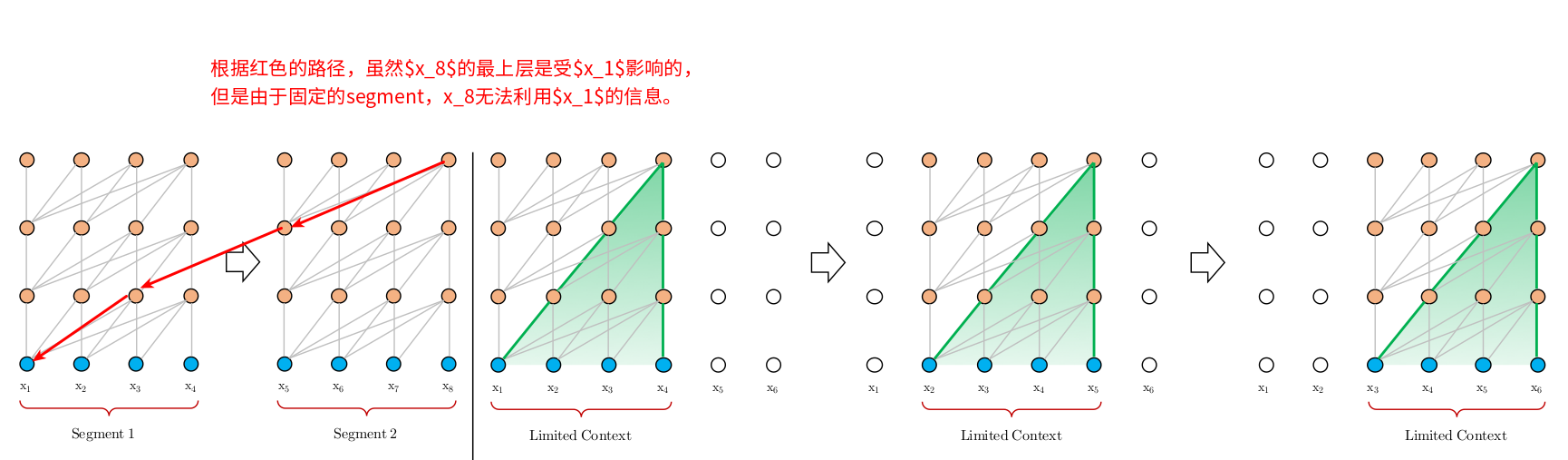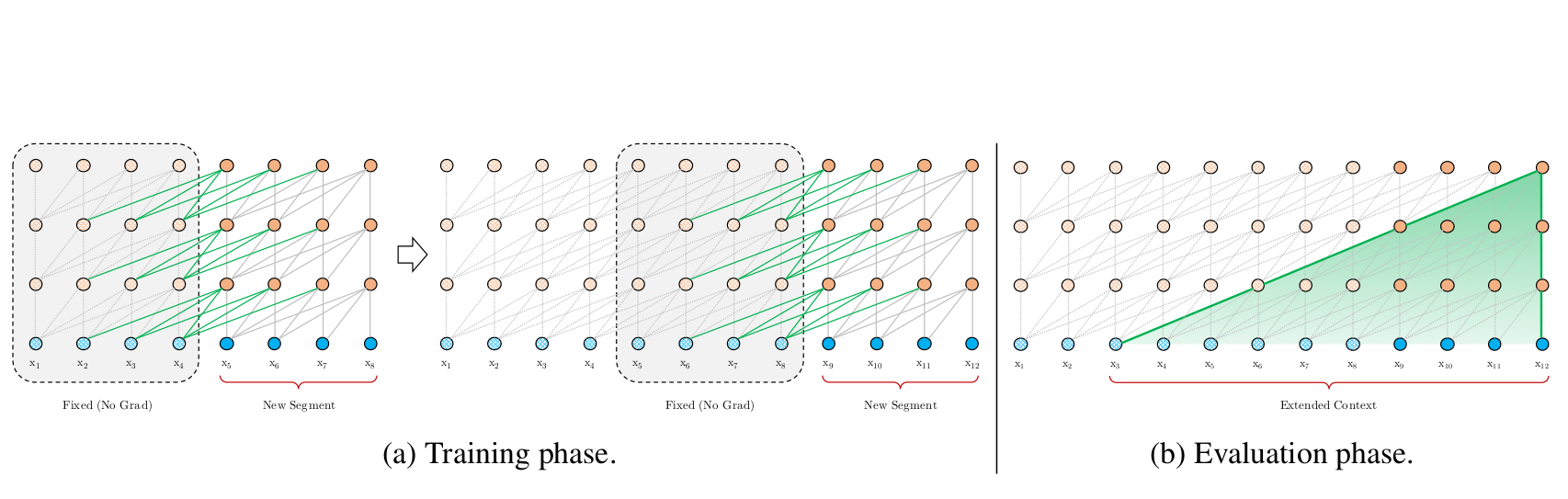### Segment基本的状态重用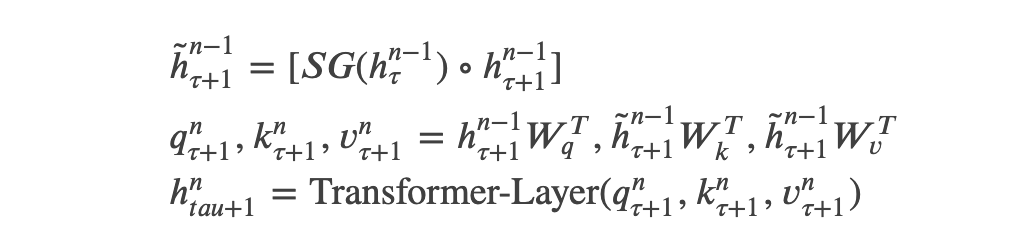### Transformer-XL的相对位置编码

Transformer-XL不能像BERT那样使用绝对位置编码，下面我们来分析一些为什么不行。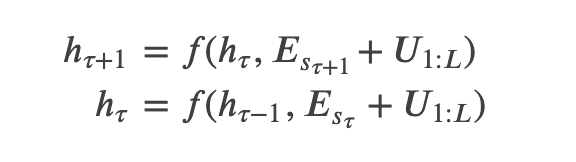### 在XLNet里融入Transformer-XL的思想

$h_{z_t}^{(m)} \leftarrow \text{Attention} (Q=h_{z_t}^{(m-1)},KV=[\tilde{h}^{(m-1)},h_{z \le t}^{(m-1)}];\theta)$

## 建模多个segment

### 相对Segment编码

BERT使用的是绝对的Segment编码，也就是第一个句子对于的Segment id是0，而第二个句子是1。这样如果把两个句子换一下顺序，那么输出是不一样的。XLNet使用的是相对的Segment编码，它是在计算Attention的时候判断两个词是否属于同一个Segment，如果位置i和j的词属于同一个segment，那么使用一个可以学习的Embedding $s_{ij}=s_+$，否则$s_{ij}=s_-$。也就是说，我们只关心它们是属于同一个Segment还是属于不同的Segment的。当我们从位置i attend to j的时候，我们会这样计算一个新的attention score：$a_{ij}=(q_i+b)^Ts_{ij}$。这里的$q_i$是第i位置的Query向量，b是一个可以学习的bias。最后我们会把这个attention score加到原来计算的Attention score里，这样它就能学到当i和j都属于某个segment的特征和i和就属于不同segment的特征。

## XLNet与BERT的对比

XLNet和BERT都是预测一个句子的部分词，但是背后的原因是不同的。BERT使用的是Mask语言模型，因此只能预测部分词(总不能把所有词都Mask了然后预测?)。而XLNet预测部分词是出于性能考虑，而BERT是随机的选择一些词来预测。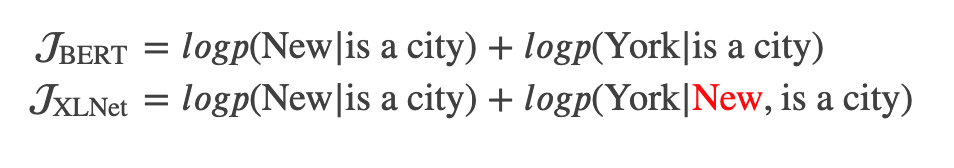## 实验

### RACE数据集

RACE数据集，它包含大概100K个问题。它是中国12岁到18岁的学生在初中和高中的英语阅读理解问题。下图是其中一个样例，我们在中学经常做的阅读理解题。

### SQuAD数据集

SQuAD是一个大规模的阅读理解任务的数据集。和前面的选择题不同，SQuAD可以看成问答题，也就是需要从阅读的文章找答案。如下图所示：

SQuAD1.1假设答案是原文的一个连续的一个或者多个词，并且答案是一定存在的。而SQuAD2.0可能会问没有答案的问题。因此为了fine-tuning SQuAD2.0，我们使用了Multi-Task Learning：其中一个损失函数是一个二分类的logistic regression损失函数，它判断有没有答案；而另一个就是标标准的span抽取的损失函数(感兴趣的读者可以参考BERT的论文)。因为v1.1的问题是包含在v2.0里的，因此在打榜的时候我们直接使用v2.0的模型去做v1.1的题目，只是把判断有没有答案的部分去掉(因为v1.1肯定有答案)。另外因为很多参赛者会使用额外的数据，我们也增加了NewsQA的数据作为训练数据。如下表所示，我们的单一的模型(很多好成绩都是好的模型的Ensembling)取得了SOTA的成绩。

GLUE数据集上的实验如下：

### ClueWeb09-B

ClueWeb09-B是一个文档排序的数据集。它主要用于搜索引擎：给定一个Query，然后给相关的网页排序。下面是实验结果：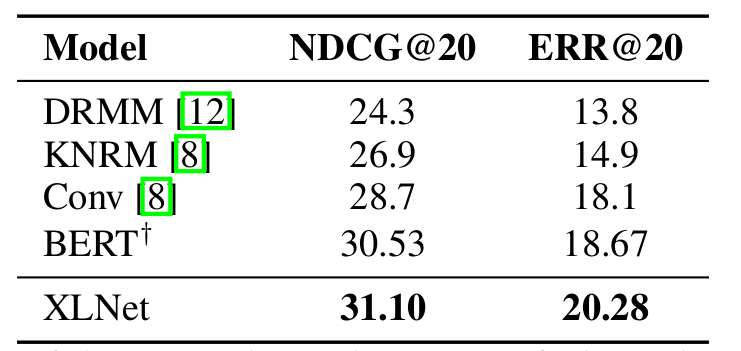### 代码